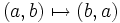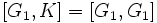# Commutator of a normal subgroup and a subgroup not implies normal

## Statement

It is possible to have a group$L$, a Normal subgroup (?)$K$ of$G$, and a subgroup$G$ of$L$, such that the Commutator of two subgroups (?)$[K,G]$ is not a normal subgroup of$L$.

## Proof

### A generic example

Let$G$ be a nontrivial perfect group. Consider the square$K = G \times G$ (the external direct product of$G$ with itself). Let$L$ be the external semidirect product of$K$ with the cyclic group of order two acting by the coordinate exchange automorphism$(a,b) \mapsto (b,a)$.

In short$L$ can also be defined as the external wreath product of$G$ and a cyclic group of order two acting regularly.

Then, define$G_1 = G \times \{ e \}$, i.e., the first direct factor of$K$, and define$G_2 = \{ e \} \times G$, i.e., the second direct factor. Observe that:

1.$K$ is normal in$L$ by construction.
2.$G_1$ is a subgroup of$L$, but is not normal in$L$, because the coordinate exchange automorphism sends$G_1$ to$G_2$.
3.$G_1$ is a direct factor of$K$, hence normal in$K$. Thus,$[G_1,K] \le G_1$.
4. On the other hand, since$G_1 \le K$,$[G_1,G_1] \le [G_1,K]$. But since$G_1 \cong G$ and$G$ is perfect, we have$G_1 = [G_1,G_1]$, so$G_1 \le [G_1,K]$.
5. Thus, by steps (3) and (4), we get$G_1 = [G_1,K]$. Step (2) tells us that$G_1$ is not normal in$L$. Thus, we have found a normal subgroup$K$ and a subgroup$G_1$ such that the commutator$[G_1,K]$ is not normal.

### Particular cases of this example

Further information: Alternating group:A5, A5 is simple, A5 is the simple non-Abelian group of smallest order

Any simple non-Abelian group is an example of a nontrivial perfect group, so the smallest particular case of this generic example is to set$G$ as the alternating group of degree five.

### A more generic example

In the above example, we assumed that$G$ was perfect. We can relax this slightly to assuming only that$G$ is non-Abelian. In this more general case, we obtain that$[G_1,K] = [G_1,G_1]$, which is a nontrivial subgroup of$L$ contained inside$G_1$. This subgroup is not normal because the coordinate exchange automorphism sends it to a corresponding subgroup of$G_2$.

This more generic example allows us some nilpotent and solvable groups:

• Setting$G$ as the symmetric group on three letters yields a group$L$ of order$6^2 \cdot 2 = 72$. This group is a solvable group, since$G$ itself is solvable.
• Setting$G$ as the dihedral group of order eight yields a group$L$ of order$8^2 \cdot 2 = 128 = 2^7$. This is a group of prime power order, and in particular, is a nilpotent group.